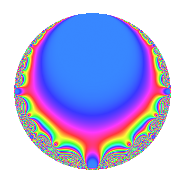Properties

 Label 8.4.bLevel 8 Weight 4 Character orbit b Rep. character $$\chi_{8}(5,\cdot)$$ Character field $$\Q$$ Dimension 2 Newforms 1 Sturm bound 4 Trace bound 0

Related objects

Defining parameters

 Level: $$N$$ = $$8 = 2^{3}$$ Weight: $$k$$ = $$4$$ Character orbit: $$[\chi]$$ = 8.b (of order $$2$$ and degree $$1$$) Character conductor: $$\operatorname{cond}(\chi)$$ = $$8$$ Character field: $$\Q$$ Newforms: $$1$$ Sturm bound: $$4$$ Trace bound: $$0$$

Dimensions

The following table gives the dimensions of various subspaces of $$M_{4}(8, [\chi])$$.

Total New Old
Modular forms 4 4 0
Cusp forms 2 2 0
Eisenstein series 2 2 0

Trace form

 $$2q$$ $$\mathstrut -\mathstrut 2q^{2}$$ $$\mathstrut -\mathstrut 12q^{4}$$ $$\mathstrut +\mathstrut 28q^{6}$$ $$\mathstrut -\mathstrut 16q^{7}$$ $$\mathstrut +\mathstrut 40q^{8}$$ $$\mathstrut -\mathstrut 2q^{9}$$ $$\mathstrut +\mathstrut O(q^{10})$$ $$2q$$ $$\mathstrut -\mathstrut 2q^{2}$$ $$\mathstrut -\mathstrut 12q^{4}$$ $$\mathstrut +\mathstrut 28q^{6}$$ $$\mathstrut -\mathstrut 16q^{7}$$ $$\mathstrut +\mathstrut 40q^{8}$$ $$\mathstrut -\mathstrut 2q^{9}$$ $$\mathstrut -\mathstrut 56q^{10}$$ $$\mathstrut -\mathstrut 56q^{12}$$ $$\mathstrut +\mathstrut 16q^{14}$$ $$\mathstrut +\mathstrut 112q^{15}$$ $$\mathstrut +\mathstrut 16q^{16}$$ $$\mathstrut -\mathstrut 28q^{17}$$ $$\mathstrut +\mathstrut 2q^{18}$$ $$\mathstrut +\mathstrut 112q^{20}$$ $$\mathstrut -\mathstrut 84q^{22}$$ $$\mathstrut -\mathstrut 304q^{23}$$ $$\mathstrut -\mathstrut 112q^{24}$$ $$\mathstrut +\mathstrut 26q^{25}$$ $$\mathstrut +\mathstrut 280q^{26}$$ $$\mathstrut +\mathstrut 96q^{28}$$ $$\mathstrut -\mathstrut 112q^{30}$$ $$\mathstrut +\mathstrut 448q^{31}$$ $$\mathstrut -\mathstrut 352q^{32}$$ $$\mathstrut +\mathstrut 168q^{33}$$ $$\mathstrut +\mathstrut 28q^{34}$$ $$\mathstrut +\mathstrut 12q^{36}$$ $$\mathstrut -\mathstrut 196q^{38}$$ $$\mathstrut -\mathstrut 560q^{39}$$ $$\mathstrut +\mathstrut 224q^{40}$$ $$\mathstrut -\mathstrut 140q^{41}$$ $$\mathstrut -\mathstrut 224q^{42}$$ $$\mathstrut +\mathstrut 168q^{44}$$ $$\mathstrut +\mathstrut 304q^{46}$$ $$\mathstrut +\mathstrut 672q^{47}$$ $$\mathstrut +\mathstrut 672q^{48}$$ $$\mathstrut -\mathstrut 558q^{49}$$ $$\mathstrut -\mathstrut 26q^{50}$$ $$\mathstrut -\mathstrut 560q^{52}$$ $$\mathstrut +\mathstrut 728q^{54}$$ $$\mathstrut -\mathstrut 336q^{55}$$ $$\mathstrut -\mathstrut 320q^{56}$$ $$\mathstrut +\mathstrut 392q^{57}$$ $$\mathstrut -\mathstrut 840q^{58}$$ $$\mathstrut -\mathstrut 672q^{60}$$ $$\mathstrut -\mathstrut 448q^{62}$$ $$\mathstrut +\mathstrut 16q^{63}$$ $$\mathstrut +\mathstrut 576q^{64}$$ $$\mathstrut +\mathstrut 1120q^{65}$$ $$\mathstrut -\mathstrut 168q^{66}$$ $$\mathstrut +\mathstrut 168q^{68}$$ $$\mathstrut +\mathstrut 448q^{70}$$ $$\mathstrut -\mathstrut 144q^{71}$$ $$\mathstrut -\mathstrut 40q^{72}$$ $$\mathstrut -\mathstrut 588q^{73}$$ $$\mathstrut +\mathstrut 1288q^{74}$$ $$\mathstrut +\mathstrut 392q^{76}$$ $$\mathstrut +\mathstrut 560q^{78}$$ $$\mathstrut -\mathstrut 928q^{79}$$ $$\mathstrut -\mathstrut 1344q^{80}$$ $$\mathstrut -\mathstrut 1510q^{81}$$ $$\mathstrut +\mathstrut 140q^{82}$$ $$\mathstrut +\mathstrut 448q^{84}$$ $$\mathstrut -\mathstrut 2324q^{86}$$ $$\mathstrut +\mathstrut 1680q^{87}$$ $$\mathstrut +\mathstrut 336q^{88}$$ $$\mathstrut +\mathstrut 532q^{89}$$ $$\mathstrut +\mathstrut 56q^{90}$$ $$\mathstrut +\mathstrut 1824q^{92}$$ $$\mathstrut -\mathstrut 672q^{94}$$ $$\mathstrut -\mathstrut 784q^{95}$$ $$\mathstrut -\mathstrut 448q^{96}$$ $$\mathstrut +\mathstrut 1988q^{97}$$ $$\mathstrut +\mathstrut 558q^{98}$$ $$\mathstrut +\mathstrut O(q^{100})$$

Decomposition of $$S_{4}^{\mathrm{new}}(8, [\chi])$$ into irreducible Hecke orbits

Label Dim. $$A$$ Field CM Traces $q$-expansion
$$a_2$$ $$a_3$$ $$a_5$$ $$a_7$$
8.4.b.a $$2$$ $$0.472$$ $$\Q(\sqrt{-7})$$ None $$-2$$ $$0$$ $$0$$ $$-16$$ $$q+(-1-\beta )q^{2}+2\beta q^{3}+(-6+2\beta )q^{4}+\cdots$$